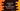# JavaScript program to print the ASCII values from A to Z alphabet## JavaScript program to print the ASCII values from A to Z

In this post, we will learn how to print the ASCII values of the alphabets in JavaScript. We will write two programs to print the ASCII values of A to Z and a to z.

### String.prototype.charCodeAt()

The String.prototype.charCodeAt() method is used to get the UTF-16 code unit of a character in a string. We can pass the index of the character to this method and it returns the UTF-16 code unit.

For example:

``````let givenString = "abcd";

console.log(givenString.charCodeAt(0));
console.log(givenString.charCodeAt(1));
console.log(givenString.charCodeAt(2));``````

It will print:

``````97
98
99``````

We can use this method to print the ASCII values of the characters from a to z. We have to pass 0 as the parameter to this method if we use it with a character.

### Method 1: By using a for loop:

We can use any loop to print the ASCII values from a to z. For example:

``````let startChar = "a";
let endChar = "z";

for (let c = startChar.charCodeAt(0); c <= endChar.charCodeAt(0); c++) {
console.log(c);
}``````

For this example, startChar is the starting character and endChar is the end character for the loop. The loop runs from the charCodeAt of startChar to charCodeAt of endChar.

If you run this program, it will print the ASCII values of a to z i.e. 97 to 122.

You can change the value of startChar and endChar values to print the ASCII values from A to Z.

``````let startChar = "A";
let endChar = "Z";

for (let c = startChar.charCodeAt(0); c <= endChar.charCodeAt(0); c++) {
console.log(c);
}``````

It will print from 65 to 90.

### Method 2: By using a while loop:

Similar to the above example, we can also use a while loop to print the ASCII values of the alphabets.

``````let startChar = "A";
let endChar = "Z";

let c = startChar.charCodeAt(0);

while (c <= endChar.charCodeAt(0)) {
console.log(c);
c++;
}``````

This is similar to the above example. The only difference is that the value of c is initialized before the loop starts and the value of c is incremented at the end of the loop.

We can change the value of startChar and endChar to print the ASCII values from A to Z.

### Method 3: With a string holding the characters:

We can also define another string to hold the characters and iterate through these characters to print the ASCII values.

``````let chars = "abcdefghijklmnopqrstuvwxyz";

for (const c of chars) {
console.log(c.charCodeAt(0));
}``````

chars string holds the lowercase characters and the for loop is printing the ASCII value of each character in that string.

We can also use a while loop:

``````let chars = "abcdefghijklmnopqrstuvwxyz";

let i = 0;

while (i < chars.length) {
console.log(chars.charAt(i).charCodeAt(0));
i++;
}``````

The value of i is initialized as 0. It will run from i = 0 to i = length of chars - 1. We are using charAt(i) to get the character at a specific position. It will give a similar result.

Or, with ES6, we can use a forEach loop:

``````let chars = "abcdefghijklmnopqrstuvwxyz";

[...chars].forEach((c) => console.log(c.charCodeAt(0)));``````